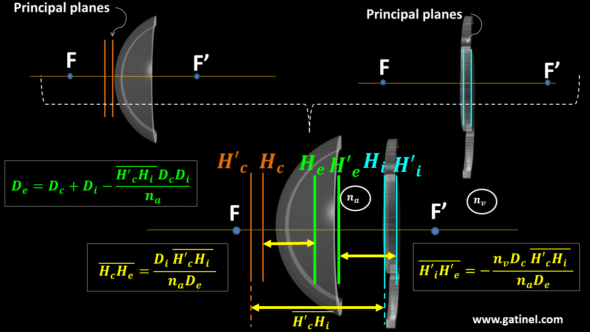+ +

# 9. Paraxial power of the thick pseudophakic eye

This page is dedicated to transforming the pseudophakic eye composed of two thick lenses (cornea and IOL) into a single element provided with its principal planes and focal distances.

If one knows the value of the paraxial constants of the cornea and the IOL (derived from their respective curvatures and thickness), one can establish, thanks to the Gullstrand formula, the optical power resulting from the couple « cornea + IOL. » This formula requires the distance between these two thick lenses: this distance is precisely that which separates the principal image plane of the cornea from the principal image plane of the intraocular lens. For numerical applications, this distance corresponds to the effective lens position (ELP).

The image focal length resulting from the combined effect of the cornea and IOL, which provides the position of the theoretical image plan off a point located at infinity, is established from the principal image plane of the pseudophakic eye.

## Pseudophakic thick lens formulas

The pseudophakic eye corresponds to a system made up of two thick elements: the cornea and the intraocular lens (IOL).

It is treated as a single system whose anterior interface is equivalent to the principal object plane of the cornea and the posterior interface the principal image plane of the IOL.

One can determine the total paraxial power (De) and the position of its principal planes (He, H’e) of the pseudophakic eye modeled with thick lenses.

We can make an analogy with a thick dioptre; the paraxial power of the anterior interface is then equated to the total power of the cornea (Dc). The power of the posterior interface is equated to the total power of the IOL (Di). Gullstrand’s formula calculates the total power of the compound pseudophakic eye and depends on the respective powers and distance between the cornea and the IOL.

In this context, the distance between these two interfaces refers to the effective lens position with thick lens elements (ELP). It does not directly depend on the anatomical distance separating these two elements (S1S3) but on the position of their respective principal planes; the ELP extends from the principal image plane of the cornea (H’c)  to the principal object plane of the implant (Hi).

We can apply the paraxial formulas that calculate the power of the pseudophakic eye assimilated to the association of the corneal and IOL using Gullstrand’s formula and determine the position of its own principal planes.Thick lens paraxial pseudophakic eye model: the optical distance separating the thick cornea and IOL refers to the effective lens position ELP). It corresponds to the distance H’cHi.

The optically relevant distance separating the two elements constitutive of the pseudophakic eye is comprised between the principal object plane of the cornea and from the principal image plane of the IOL. This distance corresponds to the ELP. The numerical values obtained depend on the values assigned to the refractive indices which separate the cornea from the IOL (refractive index of the aqueous humor: na) and from the refractive index of the vitreous (nv).

The object and image focal lengths of the pseudophakic eye are located from the position of the principal planes.

These notions are important in order to be able to move on to the next step: building a pseudophakic eye model with thick lenses and establishing the equations useful for the calculation of certain fundamental elements.

Les commentaires sont fermés.